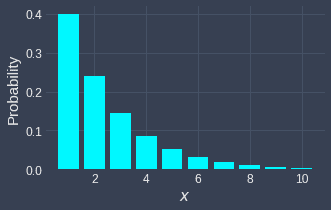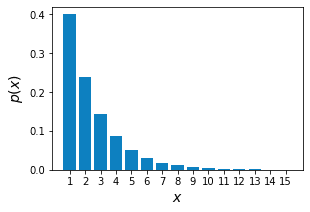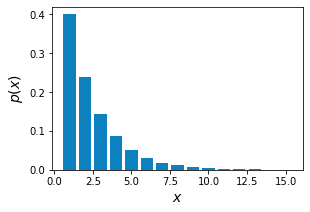search
Search
Unlock 100+ guides
search toc
close
account_circle
Profile
exit_to_app
Sign out
search
keyboard_voice
close
Searching Tips
Search for a recipe:
"Creating a table in MySQL"
Search for an API documentation: "@append"
Search for code: "!dataframe"
Apply a tag filter: "#python"
Useful Shortcuts
/ to open search panel
Esc to close search panel
to navigate between search results
d to clear all current filters
Enter to expand content previewDoc SearchCode Search BetaSORRY NOTHING FOUND!
mic
Start speaking...Voice search is only supported in Safari and Chrome.
Shrink
Navigate to

# Comprehensive Guide on Geometric Distribution

schedule Aug 12, 2023
Last updated
local_offer
Probability and Statistics
Tags
mode_heat
Master the mathematics behind data science with 100+ top-tier guides
Start your free 7-days trial now!

Before we formally define the geometric distribution, let's go through a motivating example.

# Motivating example

Suppose we repeatedly toss an unfair coin with the following probabilities:

\begin{align*} \mathbb{P}(\mathrm{H})&=0.2\\ \mathbb{P}(\mathrm{T})&=0.8\\ \end{align*}

Where $\mathrm{H}$ and $\mathrm{T}$ represent heads and tails respectively.

Let the random variable $X$ denote the first occurrence of a heads at the $X$-th trial. To obtain heads for the very first time at the $X$-th trial, we must have obtained $X-1$ number of tails before that. For instance, suppose we are interested in the probability of obtaining a heads for the first time at the 3rd trial. This means that the outcome of our tosses has to be:

$$\mathrm{T},\mathrm{T},\mathrm{H}$$

The probability of this specific outcome is:

\begin{align*} \mathbb{P}(X=3)&=(0.8)^2(0.2)^1\\ &=0.128 \end{align*}

What would the probability of obtaining a heads for the first time at the 5th trial be? In this case, the outcome of the tosses must be:

$$\mathrm{T},\mathrm{T},\mathrm{T},\mathrm{T},\mathrm{H}$$

The probability that this specific outcome occurs is:

\begin{align*} \mathbb{P}(X=5)&=(0.8)^4(0.2)^1\\ &=0.08192 \end{align*}

Hopefully you can see that, in general, the probability of obtaining a heads for the first time at the $x$-th trial is given by:

$$\mathbb{P}(X=x)=(0.8)^{x-1}(0.2)$$

Let's generalize further - instead of heads and tails, let's denote a heads as a success and a tails as a failure. If the probability of success is $p$, then the probability of failure is $1-p$. Therefore, the probability of observing a success for the first time at the $x$-th trial is given by:

$$\begin{equation}\label{eq:uBLaZ2spLI0XSW6WDoK} \mathbb{P}(X=x)=(1-p)^{x-1}\cdot{p} \end{equation}$$

Random variables that have this specific distribution is said to follow the geometric distribution.

## Assumptions of the geometric distribution

While deriving the geometric distribution, we made the following implicit assumptions:

• probability of success is constant for every trial. For our example, the probability of heads is $0.2$ for each coin toss.

• the trials are independent. For our example, the outcome of a coin toss does not affect the outcome of the next coin toss.

• the outcome of each trial is binary. For our example, the outcome of a coin toss is either heads or tails.

Statistical experiments that satisfy these conditions are called repeated Bernoulli trials.

Definition.

# Geometric distribution

A discrete random variable $X$ is said to follow a geometric distribution with parameter $p$ if and only if the probability mass function of $X$ is:

$$\mathbb{P}(X=x)=(1-p)^{x-1}\cdot{p} \;\;\;\;\;\;\; \text{for }\;x=1,2,3,\cdots$$

Where $0\le{p}\le{1}$. If a random variable $X$ follows a geometric distribution with parameter $p$, then we can write $X\sim\text{Geom}(p)$.

Example.

## Drawing balls from a bag

Suppose we randomly draw with replacement from a bag containing 3 red balls and 2 green balls until a green ball is drawn. Answer the following questions:

1. What is the probability of drawing a green ball at the 3rd trial?

2. What is the probability of drawing a green ball at or before the 3rd trial?

Solution. Let's start by confirming that this experiment is a repeated Bernoulli trials:

• probability of success, that is, drawing a green ball is constant ($p=2/5$).

• the trials are independent because we are drawing with replacement.

• the outcome is binary - we either draw a green ball or we don't.

Let $X$ be a geometric random variable representing the first time we draw a green ball at the $X$-th trial. The probability of success, that is, the probability of drawing a green ball at each trial is $p=2/5$. Therefore, the geometric probability mass function is:

$$\begin{equation}\label{eq:M8H8zn3VNWJ0gFG38kM} \mathbb{P}(X=x)=\Big(1-\frac{2}{5}\Big)^{x-1}\cdot\Big(\frac{2}{5}\Big) \end{equation}$$

The probability of drawing a green ball at the 3rd trial is:

\begin{align*} \mathbb{P}(X=3) &=\Big(1-\frac{2}{5}\Big)^{3-1}\cdot{\Big(\frac{2}{5}\Big)}\\ &=\Big(\frac{3}{5}\Big)^{2}\cdot{\Big(\frac{2}{5}\Big)}\\ &=\frac{18}{125}\\ &=0.144 \end{align*}

The probability of drawing a green ball at or before the 3rd trial is:

\begin{align*} \mathbb{P}(X\le3)&= {\color{blue}\mathbb{P}(X=3)}+ {\color{purple}\mathbb{P}(X=2)}+ {\color{orange}\mathbb{P}(X=1)}\\ &={\color{blue}\Big(1-\frac{2}{5}\Big)^2\Big(\frac{2}{5}\Big)}+ {\color{purple}\Big(1-\frac{2}{5}\Big)^1\Big(\frac{2}{5}\Big)}+ {\color{orange}\Big(\frac{2}{5}\Big)}\\ &={\color{blue}\frac{18}{125}}+ {\color{purple}\frac{6}{25}}+ {\color{orange}\frac{2}{5}}\\ &=\frac{98}{125}\\&=0.784 \end{align*}

Finally, let's graph our geometric probability mass function:We can see that $\mathbb{P}(X=3)$ is indeed roughly around $0.144$. Note that we've truncated the graph at $x=10$ but $x$ can be any positive integer.

# Properties of geometric distribution

Theorem.

## Expected value of a geometric random variable

If $X$ follows a geometric distribution with parameter $p$, then the expected value of $X$ is given by:

$$\mathbb{E}(X)=\frac{1}{p}$$

Proof. Let $X\sim\mathrm{Geom}(p)$. By the definitionlink of expected values, we have that:

\begin{equation}\label{eq:wX1Je2CfjYCv3cV1Sgl} \begin{aligned}[b] \mathbb{E}(X) &=\sum^\infty_{x=1}x\cdot\mathbb{P}(X=x)\\ &=1\cdot\mathbb{P}(X=1)+2\cdot\mathbb{P}(X=2) +3\cdot\mathbb{P}(X=3)+4\cdot\mathbb{P}(X=4)\cdots\\ &=p(1-p)^{1-1} +2p(1-p)^{2-1} +3p(1-p)^{3-1} +4p(1-p)^{4-1} +\cdots\\ &= p(1-p)^{0} +2p(1-p)^{1} +3p(1-p)^{2} +4p(1-p)^{3} +\cdots\\ &=p\Big[ 1(1-p)^{0} +2(1-p)^{1} +3(1-p)^{2} +4(1-p)^{3} +\cdots\Big]\\ &=p\Big[ {\color{orange}\sum^\infty_{i=1}i(1-p)^{i-1}}\Big] \end{aligned} \end{equation}

We now want to compute the summation:

$$\begin{equation}\label{eq:tmFosgxf7NJZupdcACh} {\color{orange}\sum^\infty_{i=1}i(1-p)^{i-1}}= 1(1-p)^{0} +2(1-p)^{1} +3(1-p)^{2} +4(1-p)^{3} +\cdots \end{equation}$$

Let's rewrite each term as:

\begin{align*} (1-p)^0&=\color{red}(1-p)^0\\ 2(1-p)^1&={\color{red}(1-p)^1}+\color{green}(1-p)^1\\ 3(1-p)^2&={\color{red}(1-p)^2}+{\color{green}(1-p)^2}+\color{blue}(1-p)^2\\ 4(1-p)^3&={\color{red}(1-p)^3}+{\color{green}(1-p)^3}+{\color{blue}(1-p)^3}+\color{purple}(1-p)^4\\ \end{align*}

To get \eqref{eq:tmFosgxf7NJZupdcACh}, we must take the summation - but the trick is to do so vertically:

\begin{align*} S_1=\sum^\infty_{i=1}(1-p)^{i-1}&={\color{red}(1-p)^0}+{\color{red}(1-p)^1}+{\color{red}(1-p)^2}+{\color{red}(1-p)^3}+\cdots\\ S_2=\sum^\infty_{i=2}(1-p)^{i-1}&={\color{green}(1-p)^1}+{\color{green}(1-p)^2}+{\color{green}(1-p)^3}+{\color{green}(1-p)^4}+\cdots\\ S_3=\sum^\infty_{i=3}(1-p)^{i-1}&={\color{blue}(1-p)^2}+{\color{blue}(1-p)^3}+{\color{blue}(1-p)^4}+{\color{blue}(1-p)^5}+\cdots \end{align*}

Notice that each of these are infinite geometric series with common ratio $(1-p)$. The only difference between them is the starting value. Because $p$ is a probability, we have that $0\lt{p}\lt1$. This also means that $0\lt{1-p}\lt1$. In our guide on geometric series, we have shownlink that all infinite geometric series converge to the following sum when the common ratio is between $-1$ and $1$:

$$\begin{equation}\label{eq:ETLEUmzvGgK9qpXwgIf} S=\frac{a}{1-r} \end{equation}$$

Where $a$ is the starting value of the series and $r$ is the common ratio. Therefore, in our case, the sum would be:

\begin{align*} S_1&=\frac{(1-p)^0}{1-(1-p)}=\frac{(1-p)^0}{p}\\ S_2&=\frac{(1-p)^1}{1-(1-p)}=\frac{(1-p)^1}{p}\\ S_3&=\frac{(1-p)^2}{1-(1-p)}=\frac{(1-p)^2}{p}\\ \end{align*}

The orange sum can therefore be written as:

\begin{align*} \color{orange}\sum^\infty_{i=1}i(1-p)^{i-1} &=S_1+S_2+S_3+\cdots\\ &=\frac{(1-p)^0}{p}+\frac{(1-p)^1}{p}+\frac{(1-p)^2}{p} +\cdots \end{align*}

Once again, we end up with yet another infinite geometric series with starting value $1/p$ and common ratio $(1-p)$. Using the formula for the sum of infinite geometric series \eqref{eq:ETLEUmzvGgK9qpXwgIf} once again gives:

\begin{equation}\label{eq:jkILy4J9QlCfNqZ0wQI} \begin{aligned}[b] \color{orange}\sum^\infty_{i=1}i(1-p)^{i-1}&=\frac{1/p}{1-(1-p)}\\ &=\frac{1/p}{p}\\ &=\frac{1}{p^2} \end{aligned} \end{equation}

Substituting \eqref{eq:jkILy4J9QlCfNqZ0wQI} into \eqref{eq:wX1Je2CfjYCv3cV1Sgl} gives:

\begin{align*} \mathbb{E}(X) &=p\Big(\frac{1}{p^2}\Big)\\ &=\frac{1}{p} \end{align*}

This completes the proof.

Theorem.

## Variance of a geometric random variable

If $X$ follows a geometric distribution with parameter $p$, then the expected value of $X$ is given by:

$$\mathbb{V}(X)=\frac{1-p}{p^2}$$

Proof. We know from the propertylink of variance that:

$$\begin{equation}\label{eq:Zfofz6F9ySPnH9Onso9} \mathbb{V}(X)=\mathbb{E}(X^2)-\big[\mathbb{E}(X)\big]^2 \end{equation}$$

We already know what $\mathbb{E}(X)$ is from earlierlink, so we have that:

$$\begin{equation}\label{eq:BFUrEk4tUwaopDxTSb9} \mathbb{V}(X)=\mathbb{E}(X^2)-\frac{1}{p^2} \end{equation}$$

We now need to derive the expression for $\mathbb{E}(X^2)$. From the definition of expected values, we have that:

\begin{equation}\label{eq:Liebvt5zDji9PljQRHJ} \begin{aligned}[b] \mathbb{E}(X^2) &=\sum^\infty_{x=1}\Big[x^2\cdot{p(1-p)^{x-1}}\Big]\\ &=\sum^\infty_{x=1}\Big[(x^2+x-x)\cdot{p(1-p)^{x-1}}\Big]\\ &=\sum^\infty_{x=1}\Big[\big[(x^2+x)-x\big]\cdot{p(1-p)^{x-1}}\Big]\\ &=\sum^\infty_{x=1}\Big[(x^2+x)\cdot{p(1-p)^{x-1}}-x\cdot{p(1-p)^{x-1}}\Big]\\ &=\sum^\infty_{x=1}\Big[(x^2+x)\cdot{p(1-p)^{x-1}}\Big] -\sum^\infty_{x=1}\Big[x\cdot{p(1-p)^{x-1}}\Big]\\ &=\Big(\sum^\infty_{x=1}x(x+1)\cdot{p(1-p)^{x-1}}\Big) -\mathbb{E}(X)\\ &=\Big(p{\color{purple}\sum^\infty_{x=1}x(x+1)(1-p)^{x-1}}\Big) -\frac{1}{p}\\ \end{aligned} \end{equation}

Now, notice how we can obtain the purple term by taking the derivative like so:

$$\begin{equation}\label{eq:ctA9d0vBqyejpCEhDWm} \frac{d}{dp}{\color{green}\sum_{x=1}^\infty(x+1)(1-p)^x} =-\color{purple}\sum_{x=1}^\infty x(x+1)(1-p)^{x-1} \end{equation}$$

Note that we are using the power rule of differentiation here.

Let's now use the properties of geometric series to find an expression for the green summation in \eqref{eq:ctA9d0vBqyejpCEhDWm}. We define a new variable $k$ such that $k=x+1$, which also means that $x=k-1$. Rewriting the green summation in terms of $k$ gives:

\begin{equation}\label{eq:QL6R8IRppv3v1qFGqed} \begin{aligned}[b] \color{green}\sum_{x=1}^\infty(x+1)(1-p)^x&= \sum_{k=2}^\infty{k(1-p)^{k-1}}\\ &=\Big((1)(1-p)^{1-1}+\sum_{k=2}^\infty{k(1-p)^{k-1}}\Big)-(1)(1-p)^{1-1}\\ &=\Big(\sum_{k=1}^\infty{k(1-p)^{k-1}}\Big)-(1)(1-p)^{1-1}\\ &=\Big(\sum_{k=1}^\infty{k(1-p)^{k-1}}\Big)-1\\ \end{aligned} \end{equation}

Now, recall that we derived the following lemma \eqref{eq:jkILy4J9QlCfNqZ0wQI} when proving the expected value earlier:

$$\begin{equation}\label{eq:BCJF0TeNFEcRdzNrRqs} {\color{orange}\sum^\infty_{i=1}i(1-p)^{i-1}} =\frac{1}{p^2} \end{equation}$$

Notice how the only difference between the summation in \eqref{eq:QL6R8IRppv3v1qFGqed} and \eqref{eq:BCJF0TeNFEcRdzNrRqs} is the symbol used. Since the symbol itself doesn't matter, we have that:

$${\color{green}\sum_{x=1}^\infty(x+1)(1-p)^x} =\frac{1}{p^2}-1$$

Taking the derivative of both sides with respective to $p$ gives:

\begin{equation}\label{eq:C5c0gN5zpfWoNmLmv79} \begin{aligned}[b] \frac{d}{dp}\color{green}\sum_{x=1}^\infty(x+1)(1-p)^x &=\frac{d}{dp}\Big(\frac{1}{p^2}-1\Big)\\ &=\frac{d}{dp}\Big(p^{-2}-1\Big)\\ &=-2p^{-3}\\ &=-\frac{2}{p^3}\\ \end{aligned} \end{equation}

Equating \eqref{eq:ctA9d0vBqyejpCEhDWm} and \eqref{eq:C5c0gN5zpfWoNmLmv79} gives:

\begin{equation}\label{eq:JMbtP2c4X0XzUrqu7Pj} \begin{aligned}[b] -{\color{purple}\sum_{x=1}^\infty x(x+1)(1-p)^{x-1}} &=-\frac{2}{p^3}\\ {\color{purple}\sum_{x=1}^\infty x(x+1)(1-p)^{x-1}} &=\frac{2}{p^3} \end{aligned} \end{equation}

Substituting \eqref{eq:JMbtP2c4X0XzUrqu7Pj} into \eqref{eq:Liebvt5zDji9PljQRHJ} gives:

\begin{equation}\label{eq:VqPsbnb96WEAsuLui4w} \begin{aligned}[b] \mathbb{E}(X^2) &=p\Big(\frac{2}{p^3}\Big)-\frac{1}{p}\\ &=\frac{2}{p^2}-\frac{1}{p}\\ &=\frac{2-p}{p^2}\\ \end{aligned} \end{equation}

Finally, substituting \eqref{eq:VqPsbnb96WEAsuLui4w} into \eqref{eq:BFUrEk4tUwaopDxTSb9} gives:

\begin{align*} \mathbb{V}(X)&= \mathbb{E}(X^2)-\frac{1}{p^2}\\ &=\Big(\frac{2-p}{p^2}\Big)-\frac{1}{p^2}\\ &=\frac{1-p}{p^2} \end{align*}

This completes the proof.

Theorem.

## Cumulative distribution function of the geometric distribution

The cumulative distribution function of the geometric distribution with success probability $p$ is given by:

$$F(x)=\mathbb{P}(X\le{x})=1-(1-p)^x$$

Proof. We use the definition of cumulative distribution and geometric distribution:

\begin{align*} F(x)&= \mathbb{P}(X\le{x})\\ &=\sum^x_{i=1}\mathbb{P}(X=i)\\ &=\sum^x_{i=1}p(1-p)^{i-1}\\ &=p(1-p)^{0}+p(1-p)^{1}+p(1-p)^{2}+\cdots+p(1-p)^{x-1}\\ \end{align*}

Notice that this is a finite geometric series with starting value $p$ and common ratio $(1-p)$. Using the formulalink for the sum of a finite geometric series, we have that:

\begin{align*} F(x) &=\frac{(p)(1-(1-p)^{x})}{1-(1-p)}\\ &=\frac{p(1-(1-p)^{x})}{p}\\ &=1-(1-p)^{x}\\ \end{align*}

This completes the proof.

Theorem.

## Memoryless property of the geometric distribution

The geometric distribution satisfies the memoryless property, that is:

$$\mathbb{P}(X\gt{m+n}\;|\;X\gt{n})=\mathbb{P}(X\gt{m})$$

Where $m$ and $n$ are non-negative integers. Note that the geometric distribution is the only discrete probability distribution with the memoryless property.

Intuition. Suppose we keep tossing a coin until we observe our first heads. We know that if we let random variable $X$ represent the outcome of heads at the $X$-th trial, then $X$ is a geometric random variable with success probability $p$. Suppose we are interested in the probability that we get heads for the first time after trial $5$, that is:

$$\begin{equation}\label{eq:ooYrmktgCHfXpKBZwTL} \mathbb{P}(X\gt5) \end{equation}$$

Now, suppose we have already observed more than $2$ tails. We can update our probability \eqref{eq:ooYrmktgCHfXpKBZwTL} to include this information:

$$\mathbb{P}(X\gt5\;\vert\;X\gt2)$$

We now use the formula for conditional probability:

$$\mathbb{P}(X\gt5\;\vert\;X\gt2)= \frac{\mathbb{P}(X\gt5\;\text{and}\;X\gt2)}{\mathbb{P}(X\gt2)}$$

Notice how $\mathbb{P}(X\gt5\;\text{and}\;X\gt2)$ is equal to $\mathbb{P}(X\gt5)$ because when $X\gt5$, then $X\gt2$ is always true. Therefore, we have that:

$$\begin{equation}\label{eq:XDyz0O6555xlpibL8kt} \mathbb{P}(X\gt5\;\vert\;X\gt2)= \frac{\mathbb{P}(X\gt5)}{\mathbb{P}(X\gt2)} \end{equation}$$

To calculate the two probabilities, we can use the geometric cumulative distribution functionlink that we derived earlier:

$$\begin{equation}\label{eq:LftighdPaL5ZDFcm1P1} \mathbb{P}(X\le{x})=1-(1-p)^x \end{equation}$$

Taking the complement on both sides:

\begin{equation}\label{eq:DVNJ1bsgANPTzCUJUqP} \begin{aligned}[b] 1-\mathbb{P}(X\le{x})&=1-[1-(1-p)^x]\\ \mathbb{P}(X\gt{x})&=(1-p)^x \end{aligned} \end{equation}

Therefore, $\mathbb{P}(X\gt5)$ and $\mathbb{P}(X\gt2)$ in \eqref{eq:XDyz0O6555xlpibL8kt} can be expressed as:

\begin{align*} \mathbb{P}(X\gt5\;\vert\;X\gt2) &=\frac{(1-p)^5}{(1-p)^2}\\ &=(1-p)^3 \end{align*}

We can simplify this further using \eqref{eq:DVNJ1bsgANPTzCUJUqP} again:

$$\mathbb{P}(X\gt5\;\vert\;X\gt2) =\mathbb{P}(X\gt3)$$

This means that the probability of observing a heads after the $5$-th trial given that we have already observed $2$ tails is equal to the probability of starting over and observing the first heads after $3$ trials. This makes sense because the past outcomes ($2$ tails in this case) do not affect subsequent outcomes, and hence we can forget about them and act as if we're starting a new coin-toss experiment with the remaining number of trials ($3$ in this case).

Proof. The proof of the memoryless property follows the same logic. Consider a geometric random variable $X$ with probability of success $p$. Recall from earlier that the probability of the first heads occurring after the $5$-th trial given that we have already observed $2$ tails is:

$$\mathbb{P}(X\gt5\;\vert\;X\gt2)= \frac{\mathbb{P}(X\gt5\;\text{and}\;X\gt2)}{\mathbb{P}(X\gt2)}$$

Instead of using these concrete numbers, we replace $5$ with $m+n$ and $2$ with $n$.

\begin{align*} \mathbb{P}(X\gt{m+n}\;\vert\;X\gt{n}) &=\frac{\mathbb{P}(X\gt{m+n}\;\text{and}\;X\gt{n})}{\mathbb{P}(X\gt{n})}\\ &=\frac{\mathbb{P}(X\gt{m+n})}{\mathbb{P}(X\gt{n})}\\ &=\frac{(1-p)^{m+n}}{(1-p)^n}\\ &=(1-p)^{m}\\ &=\mathbb{P}(X\gt{m})\\ \end{align*}

This completes the proof.

Theorem.

# Alternate parametrization of the geometric distribution

A discrete random variable $X$ is also said to follow a geometric distribution with parameter $p$ if the probability mass function of $X$ is:

$$\mathbb{P}(X=x)=(1-p)^x\cdot{p} \;\;\;\;\;\;\; \text{for }\;x=0,1,2,\cdots$$

Where $0\le{p}\le{1}$.

Intuition and proof. We have introduced the geometric random variable $X$ as observing the first success at the $X$-th trial. The probability mass function of $X$ was derived to be:

$$\begin{equation}\label{eq:Wm4Wx5OQCKTzNXbJwwW} \mathbb{P}(X=x)=(1-p)^{x-1}\cdot{p} \end{equation}$$

Where $p$ is the probability of success and $x=1,2,3,\cdots$.

There exists an equivalent formulation of the geometric distribution where we let random variable $X$ represent the number of failures before the first success. The key is to notice that observing the first success at the $X$-th trial is logically equivalent to observing $X-1$ failures before the first success. For instance, observing the first success at the $5$-th trial is the same as observing $5-1=4$ failures before the first success:Let's go the other way now - if we let random variable $X$ represent the number of failures before the first success, then we must observe the first success at the $(X+1)^\text{th}$ trial. We know that $X+1$ follows a geometric distribution with probability mass function:

$$\begin{equation}\label{eq:sTwVrvAjmdD3HLG0aA0} \mathbb{P}(X+1=x+1)=(1-p)^{(x+1)-1}\cdot{p} \end{equation}$$

Let's simplify the left-hand side:

$$\mathbb{P}(X+1=x+1)= \mathbb{P}(X=x)$$

Next, we simplify the right-hand side:

$$(1-p)^x\cdot{p}$$

Therefore, \eqref{eq:sTwVrvAjmdD3HLG0aA0} is:

$$\mathbb{P}(X=x)= (1-p)^x\cdot{p}$$

Finally, since $X$ represents the number of failures before the first success, $X$ can take on the values $X=0,1,2,\cdots$. This is slightly different from what $X$ can take on in the original definition of the geometric distribution, which was $X=1,2,3,\cdots$. This completes the proof.

# Working with geometric distribution using Python

## Computing probabilities

Consider the example from earlier:

Suppose we randomly draw with replacement from a bag containing 3 red balls and 2 green balls until a green ball is drawn. What is the probability of drawing a green ball at the 3rd trial?

If we define random variable $X$ as the number of trials needed to observe the first green ball at the $X$-th trial, then $X\sim\text{Geom}(2/5)$. We then use the geometric probability mass function to compute the probability of $X=3$:

\begin{align*} \mathbb{P}(X=3) &=\Big(1-\frac{2}{5}\Big)^{3-1}\cdot{\Big(\frac{2}{5}\Big)}\\ &=0.144 \end{align*}

Instead of computing the probability by hand, we can use Python's SciPy library:

 from scipy.stats import geomx = 3p = (2/5)geom.pmf(x, p) 0.144 

Notice that the computed result is identical to the hand-calculated result.

## Drawing probability mass function

Suppose we wanted to draw the probability mass function of $X\sim\text{Geom}(2/5)$:

$$\mathbb{P}(X=x)=\Big(1-\frac{2}{5}\Big)^{x-1}\cdot\Big(\frac{2}{5}\Big) \;\;\;\;\;\;\; \text{for }\;x=1,2,3,\cdots$$

We can call the geom.pdf(~) function on a list of positive integers:

 import matplotlib.pyplot as pltp = (2/5)n = 15xs = list(range(1, n+1)) # [0,1,2,...,15]pmfs = geom.pmf(xs, p)str_xs = [str(x) for x in xs] # Convert list of integers into list of string labelsplt.bar(str_xs, pmfs)plt.xlabel('$x$')plt.ylabel('$p(x)$')plt.show() 

This generates the following plot:Note that we converted the list of integers into a list of string labels, otherwise the $x$-axis will contain decimals:thumb_up
thumb_down
Comment
Citation
Ask a question or leave a feedback...
thumb_up
0
thumb_down
0
chat_bubble_outline
0
settings
Enjoy our search
Hit / to insta-search docs and recipes!